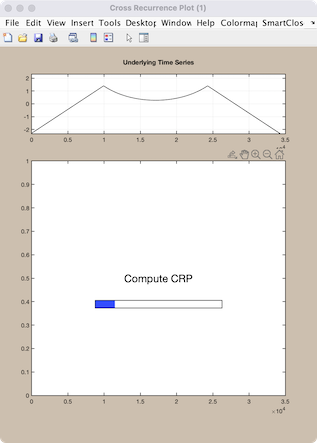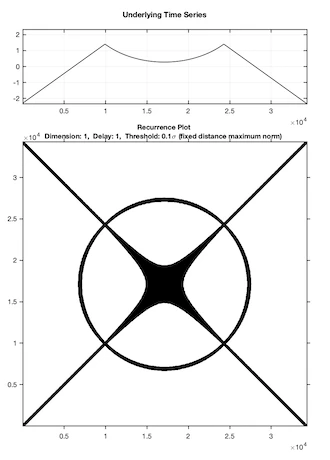# CRP Toolbox

## crp_big

Creates a cross recurrence plot/ recurrence plot or a distance matrix/ order matrix..

### Syntax

``````crp_big(x)
crp_big(x,y)
crp_big(x,m,t,e)
r=crp(x,[],m,t,e)
r=crp(x,m,t,e,'param1','param2',...)
r=crp(x,y,m,'param1')
``````

### Description

Creates a cross recurrence plot/ recurrence plot. In contrast to CRP, long data series can be used. Results can be stored into the workspace.

Allows to change the parameters interactively by using a GUI.

The source-data x and test-data y can be one- or a two-coloumn vectors (then, in the first column have to be the time); if the test-data y is not specified, a simple (auto) recurrence plot is created.

### Parameters

Dimension m, delay t and the size of neighbourhood e are the first three numbers after the data series; further parameters can be used to switch between various methods of finding the neighbours of the phasespace trajectory, to suppress the normalization of the data and to suppress the GUI (useful in order to use this programme by other programmes).

 Methods of finding the neighbours/ of plot. 'maxnorm' - Maximum norm. 'euclidean' - Euclidean norm. 'minnorm' - Minimum norm. 'nrmnorm' - Euclidean norm between normalized vectors(all vectors have the length one). 'rr' - Maximum norm, fixed recurrence rate. 'fan' - Fixed amount of nearest neighbours. 'inter' - Interdependent neighbours. 'omatrix' - Order matrix. 'opattern' - Order patterns recurrence plot. 'distance' - Distance coded matrix (global CRP, Euclidean norm). Normalization of the data series. 'normalize' - Normalization of the data. 'nonormalize' - No normalization of the data. Suppressing the GUI. 'gui' - Creates the GUI and the output plot. 'nogui' - Suppresses the GUI and the output plot. 'silent' - Suppresses all output.

Parameters are optional and not required.

### Limitations

In contrast to crp and crp2, this command allows to work with longer data series. The algorithm computes the CRP piecewise. However, the possibility to store the CRP in the workspace limits the length od data series again. However, a solution for using long data series you can find under the description for crp.

### Examples

``````a=sqrt(100^2-(-71:71).^2); b=1:100;
b(101:100+length(a))=-(a)+170;
b(end+1:end+100)=100:-1:1;
c=resample(b,10,1); % resample to mimic long time series
crp_big(c,1,1,.1,'euclidean')
``````